## Average: Formulas, Tricks, Examples and Online Test

Average refers to the sum of numbers divided by n. Also called the mean average.

Sums of data divided by the number of items in the data will give the mean average. The mean average is used quite regularly to determine final math marks over a term or semester. Averages are often used in sports: batting averages which means number of hits to number of times at bat. Gas mileage is determined by using averages.

Hence, average =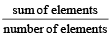For Example: To find the average of 3, 5 and 7.

Solution

Step 1: Find the sum of the numbers.

3 + 5 + 7 = 15

Step 2: Calculate the total number.

There are 3 numbers.

Step 3: Finding average 15/3 = 5

### Quicker Method to Solve the Questions

Sum of elements = average × no. of elements

Example 1: The average of marks obtained by 4 students in a class is 65. Find the sum of marks obtained?
Solution. Here, number of marks obtained = 4

Average = 65

∴ sum of marks obtained = 65 × 4 = 260

Number of elements =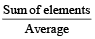Example 2: If the sum of elements and average are respectively 65 and 13, then find the number of elements.

Solution. Number of elements ==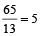Average of a group consisting of two different groups whose averages are known:
Let a group with average a contain m quantities and another group of n quantities whose average is b, then the average of group c containing at a + b quantities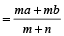Example 3: There are 30 students in a class. The average age of the first 10 students is 12.5 years. The average age of the next 20 students is 13.1 years. Find the average age of the whole class.

Solution.Total age of 10 students = 12.5 × 10 = 125 years

Total age of 20 students = 13.1 × 20 = 262 years

∴ Average age of 30 students =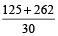=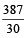= 12.9 years

If in a group one or more new quantities are added or excluded, then new quantity or sum.
= [change in no. of quantities × original average] ±
[change in average × final no. of quantities]
Take + ve sign if quantities added and take –ve sign if quantities removed

Example 4: The average weight of 24 students in a class is 35 kg. if the weight of the teacher is included, the average weight rises by 400 gms. find the weight of the teacher.

Solution.Total weight of 24 students = (24 × 35) kg = 840 kg

Total weight of 24 students and the teacher = (25 × 35.4) kg = 885 kg

∴ Weight of teacher = (885 – 840) kg = 45 kg